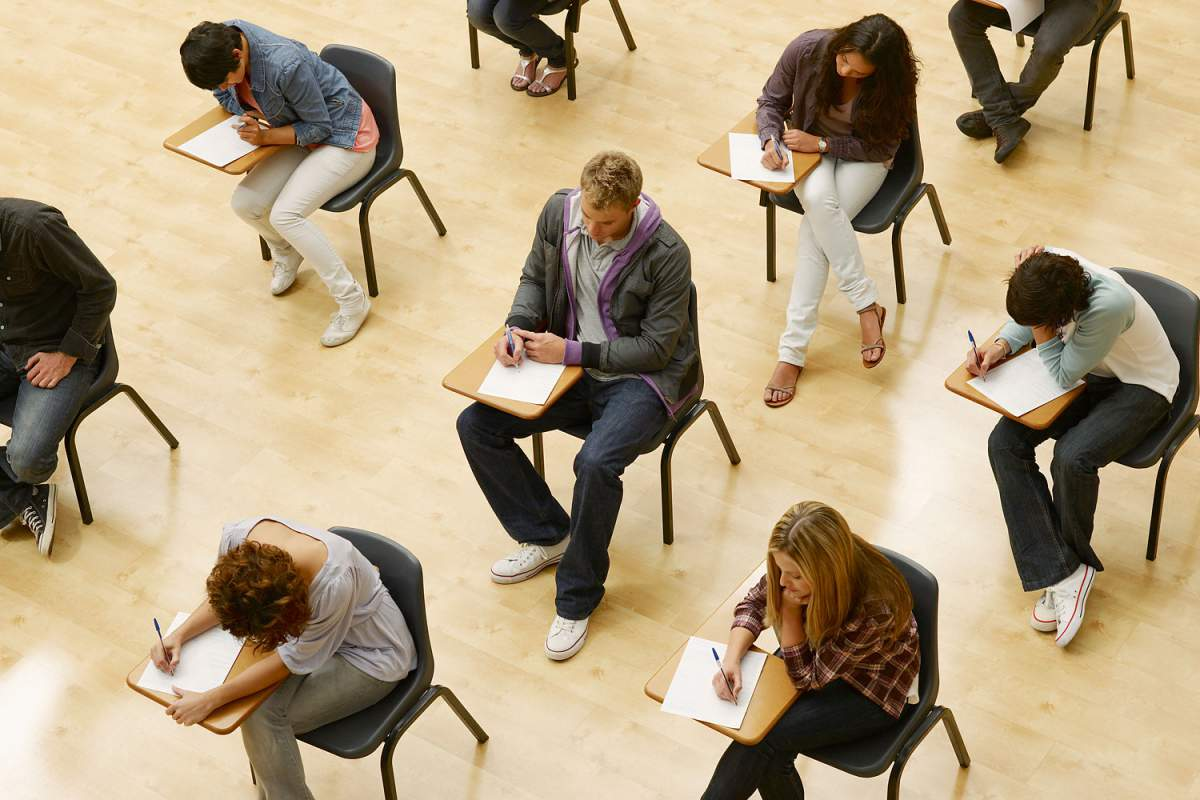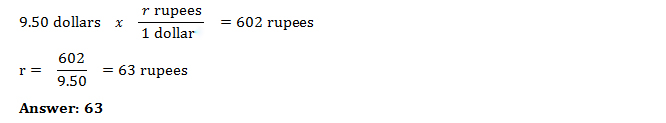|
|

Solve Real World Problems on New SAT Math Test

Students will have to show the capacity for sustained reasoning over the multiple steps required to answer many of the SAT math questions.
BY Braingain Staff Writer |   06-04-2015

Will the new SAT be easier than the old one? It's time to find out by taking a stab at the sample questions published by College Board. The math ones look fairly different from traditional SAT questions. Interestingly, College Board's sample math questions are firmly grounded in real-world contexts.

If you are sitting for the redesigned SAT exam in 2016 or later, take a crack at solving this math problem. It shows how students might be asked to demonstrate algebraic, proportional, and quantitative reasoning while solving real-world problems on the redesigned SAT.

SAT Sample Math QuestionAn international bank issues its Traveler credit cards worldwide. When a customer makes a purchase using a Traveler card in a currency different from the customer’s home currency, the bank converts the purchase price at the daily foreign exchange rate and then charges a 4% fee on the converted cost.

Sara lives in the United States, but is on vacation in India. She used her Traveler card for a purchase that cost 602 rupees (Indian currency). The bank posted a charge of \$9.88 to her account that included the 4% fee.

Part 1

What foreign exchange rate, in Indian rupees per one U.S. dollar, did the bank use for Sara’s charge? Round your answer to the nearest whole number.

Part 2

A bank in India sells a prepaid credit card worth 7,500 rupees. Sara can buy the prepaid card using dollars at the daily exchange rate with no fee, but she will lose any money left unspent on the prepaid card. What is the least number of the 7,500 rupees on the prepaid card Sara must spend for the prepaid card to be cheaper than charging all her purchases on the Traveler card? Round your answer to the nearest whole number of rupees.

Solution

Part 1: \$9.88 represents the conversion of 602 rupees plus a 4% fee on the converted cost.

To calculate the original cost of the item in dollars, x:

1.04x = 9.88

x = 9.5

Since the original cost is \$9.50, to calculate the exchange rate r, in Indian rupees per one U.S. dollar:Part 2:

Let d dollars be the cost of the 7,500-rupee prepaid card. This implies that the exchange rate on this particular day is d/7,500 dollar per rupee. Suppose Sara’s total purchases on the prepaid card were r rupees. The value of the r rupees in dollars is ( d/7,500 ) r dollars. If Sara spent the r rupees on the Traveler card instead, she would be charged (1.04) (d/7,500 ) r dollars. To answer the question about how many rupees Sara must spend in order to make the Traveler card a cheaper option (in dollars) for spending the r rupees, we set up the inequality 1.04 (d/7,500 ) rd.

Rewriting both sides reveals 1.04 (r /7,500) d ≥ (1)d, from which we can infer 1.04 (r/7,500 ) ≥ 1. Dividing on both sides by 1.04 and multiplying on both sides by 7,500, finally yields r ≥ 7,212. Hence the least number of rupees Sara must spend for the prepaid card to be cheaper than the Traveler card is 7,212.

Example 2 is an extended-thinking question that requires students to go through multiple steps to produce the solution. Such extended-thinking questions on the redesigned SAT require students to deeply think through the process of solving problems. Set within a range of real-world contexts, extended-thinking questions require students to make sense of problems and persevere in solving them; make connections between and among the different parts of a stimulus; plan a solution approach, as no scaffolding is provided to suggest a solution strategy; abstract, analyze, and refine an approach as needed; and produce and validate a response. These types of questions require the application of complex cognitive skills and will require a little more thinking time for students. Students will generally be seeing longer problems in their postsecondary work. By including extended thinking questions, the redesigned SAT rewards and incentivizes aligned, productive work in classrooms.

SAT Math Content

You may now exhale! There are still hard questions on the math, some of them testing concepts left out of the old SAT, such as basic trigonometry. Ninety percent of the test consists of three content areas: Heart of Algebra, Problem Solving and Data Analysis, and Passport to Advanced Math. The first two areas cover algebra and data analysis skills, namely linear equations, functions, ratios, percents, and proportions. Passport to Advanced Math covers complex equations, including quadratics and their applications in science and social science.  The remaining 10 percent of the exam comprises plane geometry.

Limits on Calculator Use

There are two math sections, a short one during which examinees cannot use a calculator, followed by a long one (45 minutes) during which they can. Emma Chomin at Veritas Prep highlighted in Bloomberg that the calculator section will include “questions in which the calculator could be a deterrent to expedience, thus assessing appropriate use of tools.”

The Math test will include 45 multiple-choice questions in total, which will each have four answer choices rather than the current five. One of the biggest changes is that the 0.25 points penalty for wrong answers, which discouraged students from guessing, will be eliminated. Students will instead receive a point for each question they answer correctly.

The good news on the math is that the new format tests fewer questions and goes deeper on them, so students may no longer need to focus on abstruse stuff such as permutations and combinations.

Name:

Email:x